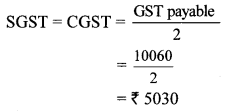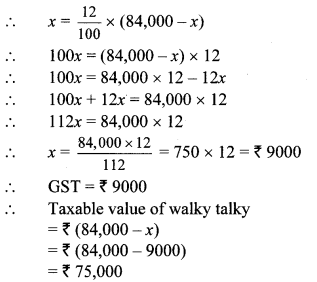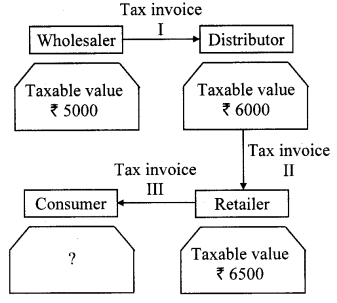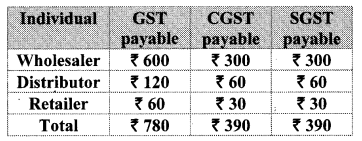# Maharashtra Board 10th Class Maths Part 1 Problem Set 4A Solutions Chapter 4 Financial Planning

Balbharti Maharashtra State Board Class 10 Maths Solutions covers the Problem Set 4A Algebra 10th Class Maths Part 1 Answers Solutions Chapter 4 Financial Planning.

## Problem Set 4A Algebra 10th Std Maths Part 1 Answers Chapter 4 Financial Planning

Financial Planning Class 10 Problem Set 4a Question 1.
Write the correct alternative for each of the following.

i. Rate of GST on essential commodities is ______
(A) 5%
(B) 12%
(C) 0%
(D) 18%
(C)

ii. The tax levied by the central government for trading within state is ______
(A) IGST
(B) CGST
(C) SGST
(D) UTGST
(B)

iii. GST system was introduced in our country from ______
(A) 31st March 2017
(B) 1st April 2017
(C) 1st January 2017
(D) 1st July 2017
(D)

iv. The rate of GST on stainless steel utensils is 18%, then the rate of state
GST is ______
(A) 18%
(B) 9%
(C) 36%
(D) 0.9%
(B)

v. In the format of GSTIN there are ______ alpha-numerals.
(A) 15
(B) 10
(C) 16
(D) 9
(A)

vi. When a registered dealer sells goods to another registered dealer under GST, then this trading is termed as ______
(A) BB
(B) B2B
(C) BC
(D) B2C
(B)

10th Class Algebra Problem Set 4a Question 2.
A dealer has given 10% discount on a showpiece of ₹ 25,000. GST of 28% was charged on the discounted price. Find the total amount shown in the tax invoice. What is the amount of CGST and SGST.
Solution:
Printed price of showpiece = ₹ 25,000,
Rate of discount = 10%
∴ Amount of discount = 10% of printed price
= $$\frac { 10 }{ 100 }$$ × 25000
= ₹ 2500
∴ Taxable value
= Printed price – discount
= 25,000 – 2500 = ₹ 22,500
Rate of GST = 28%
∴ Rate of CGST = 14% and
Rate of SGST = 14%
CGST = 14% of taxable value
= $$\frac { 14 }{ 100 }$$ × 22500
= ₹ 3150
∴ CGST = SGST = ₹ 3150
∴ Total amount of tax invoice
= Taxable value + CGST + SGST
= 22500 + 3150 + 3150
= ₹ 28,800
∴ The total amount shown in the tax invoice is ₹ 28,800, and the amount of CGST and SGST is ₹ 3150 each.

Financial Planning Problem Set 4a Question 3.
A ready-made garment shopkeeper gives 5% discount on the dress of ₹ 1000 and charges 5% GST on the remaining amount, then what is the purchase price of the dress for the customer?
Solution:
Printed price of dress = ₹ 1000
Rate of discount = 5%
∴ Amount of discount = 5% of printed price
= $$\frac { 5 }{ 100 }$$ × 1000
= ₹ 50
∴ Taxable value = Printed price – discount
= 1000 – 50
= ₹ 950
Rate of GST = 5%
∴ GST = 5% of taxable value
= $$\frac { 5 }{ 100 }$$ × 950
∴ GST = ₹ 47.5
Purchase price of the dress
= Taxable value + GST
= 950 + 47.5 = ₹ 997.50
∴ Purchase price of the dress for the customer is ₹ 997.50.

Question 4.
A trader from Surat, Gujarat sold cotton clothes to a trader in Rajkot, Gujarat. The taxable value of cotton clothes is ₹ 2.5 lacs. What is the amount of GST at 5% paid by the trader in Rajkot?
Solution:
Taxable amount of cotton clothes = ₹ 2.5 lacs,
Rate of GST = 5%
GST = 5% of taxable amount
= $$\frac { 5 }{ 100 }$$ × 2,50,000
= ₹ 12500
∴ Trader of Rajkot has to pay GST of ₹ 12,500.

Question 5.
Smt. Malhotra purchased solar panels for the taxable value of ₹ 85,000. She sold them for ₹ 90,000. The rate of GST is 5%. Find the ITC of Smt. Malhotra. What is the amount of GST payable by her?
Solution:
Output tax = 5% of 90000
= $$\frac { 5 }{ 100 }$$ × 90000
= ₹ 4500
Input tax = 5% of 85000
= $$\frac { 5 }{ 100 }$$ × 85000
= ₹ 4250
ITC = ₹ 4250.
∴ GST payable = Output tax – ITC
= 4500 – 4250
GST payable = ₹ 250
∴ ITC of Smt. Malhotra is ₹ 4250 and amount of GST payable by her is ₹ 250.

Question 6.
A company provided Z-security services for the taxable value of ₹ 64,500. Rate of GST is 18%. Company had paid GST of ₹ 1550 for laundry services and uniforms etc. What is the amount of ITC (input Tax Credit)? Find the amount of CGST and SGST payable by the company.
Solution:
Output tax = 18% of 64500
= $$\frac { 18 }{ 100 }$$ × 64500
= ₹ 11610
Input tax = ₹ 1550
GST payable = Output tax – ITC
= 11610 – 1550
∴ GST payable = ₹ 10060∴ Amount of ITC is ₹ 1550. Amount of CGST and SGST payable by the company is ₹ 5030 each.

Question 7.
A dealer supplied Walky-Talky set of ₹ 84,000 (with GST) to police control room. Rate of GST is 12%. Find the amount of state and central GST charged by the dealer. Also find the taxable value of the set.
Solution:
Let the amount of GST be ₹ x.
Price of walky talky with GST = ₹ 84,000
Taxable value of walky talky = ₹ (84,000 – x)
Now, GST = 12% of taxable value∴ Amount of state and central GST charged by the dealer is ₹ 4,500 each. Taxable value of the set is ₹ 75,000.

Question 8.
A wholesaler purchased electric goods for the taxable amount of ₹ 1,50,000. He sold it to the retailer for the taxable amount of ₹ 1,80,000. Retailer sold it to the customer for the taxable amount of ₹ 2,20,000. Rate of GST is 18%. Show the computation of GST in tax invoices of sales. Also find the payable CGST and payable SGST for wholesaler and retailer.
Solution:
For Wholesaler:
Output tax = 18% of ₹ 1,80,000Statement of GST payable at each stage of trading:Question 9.
Anna Patil (Thane, Maharashtra) supplied vacuum cleaner to a shopkeeper in Vasai (Mumbai) for the taxable value of ₹ 14,000, and GST rate of 28% . Shopkeeper sold it to the customer at the same GST rate for ₹ 16,800 (taxable value). Find the following:
i. Amount of CGST and SGST shown in the tax invoice issued by Anna Patil.
ii. Amount of CGST and SGST charged by the shopkeeper in Vasai.
iii. What is the CGST and SGST payable by shopkeeper in Vasai at the time of filing the return.
Solution:
i. For Anna Patil:
Output tax = 28% of 14,000
= $$\frac { 18 }{ 100 }$$ × 14000
= ₹ 3920
∴ CGST = SGST = $$\frac { GST }{ 2 }$$
= $$\frac { 3920 }{ 2 }$$
= ₹ 1960
∴ Amount of CGST and SGST shown in the tax invoice issued by Anna Patil is ₹ 1960 each.

ii. For Shopkeeper in Vasai:
Output tax = 28% of 16,800
= $$\frac { 28 }{ 100 }$$ × 16,800
= ₹ 4704
∴ CGST = SGST = $$\frac { GST }{ 2 }$$
= $$\frac { 4704 }{ 2 }$$
= ₹ 2352
∴ Amount of CGST and SGST charged by the shopkeeper in Vasai is ₹ 2352 each.

iii. ITC = ₹ 3920
GST payable by shopkeeper in Vasai
= Output tax – ITC
= 4704 – 3920
= ₹ 784∴ CGST and SGST payable by shopkeeper in Vasai at the time of filing the return is ₹ 392 each.

Question 10.
For the given trading chain prepare the tax invoice I, II, III. GST at the rate of 12% was charged for the article supplied.i. Prepare the statement of GST payable under each head by the wholesaler, distributor and retailer at the time of filing the return to the government.
ii. At the end what amount is paid by the consumer?
iii. Write which of the invoices issued are B2B and B2C.
Solution:
i. For wholesaler:
Output tax = 12% of 5000
= $$\frac { 12 }{ 100 }$$ × 5000 = ₹ 600
For Distributor:
Output Tax = 12% of 6000
= $$\frac { 12 }{ 100 }$$ × 6000 = ₹ 720
ITC = ₹ 600
∴ GST payable = Output tax – ITC
= 720 – 600
= ₹ 120
For Retailer:
Output tax = 12 % of 6500
= $$\frac { 12 }{ 100 }$$ × 6500 = ₹ 780
ITC = ₹ 720
∴ GST payable = Output tax – ITC
= 780 – 720 = ₹ 60
Statement of GST payable at each stage of trading:ii. ITC for consumer = ₹ 780
∴ Amount paid by consumer
= taxable value + ITC
= 6500 + 780
= ₹ 7280
∴ Amount paid by the consumer is ₹ 7280.

iii. B2B = Wholesaler to Distributor
B2B = Distributor to Retailer
B2C = Retailer to Consumer

Scroll to Top